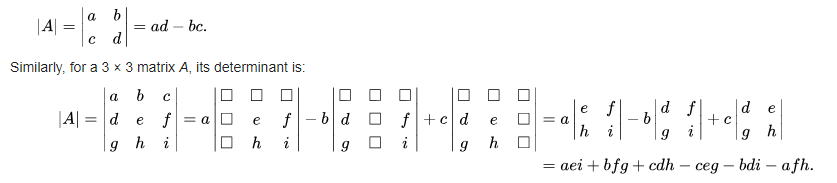﻿ NumPy: Compute the determinant of an array - w3resource# NumPy: Compute the determinant of an array

## NumPy: Linear Algebra Exercise-11 with Solution

Write a NumPy program to compute the determinant of an array.

From Wikipedia: In linear algebra, the determinant is a value that can be computed from the elements of a square matrix. The determinant of a matrix A is denoted det(A), det A, or |A|. Geometrically, it can be viewed as the scaling factor of the linear transformation described by the matrix.Sample Solution :

Python Code :

``````import numpy as np
a = np.array([[1,2],[3,4]])
print("Original array:")
print(a)
result =  np.linalg.det(a)
print("Determinant of the said array:")
print(result)
``````

Sample Output:

```Original array:
[[1 2]
[3 4]]
Determinant of the said array:
-2.0000000000000004
```

Python Code Editor:

Have another way to solve this solution? Contribute your code (and comments) through Disqus.

What is the difficulty level of this exercise?

Test your Python skills with w3resource's quiz

﻿

## Python: Tips of the Day

```print(2_000_000)
```2000000